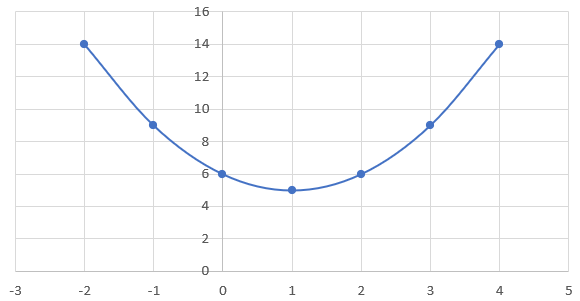# Completing the Square

Completing the Square is the process of turning a quadratic function in Standard Form ($$ax^2 + bx + c$$) into:

$$a(x - h)^2 + k$$

• $$x$$ represents the dependent variable
• $$d$$ represents the x-coordinate of the vertex
• $$e$$ represents the y-coordinate of the vertex

The core advantage to Completing the Square is that you are able to determine the vertex of the parabola by identifying the $$h$$ and $$k$$ values. For example, we can determine that the vertex of the function $$(x - 1)^2 + 5$$ is $$(1, 5)$$ since $$h = 1$$ and $$k = 5$$. This function can be graphed as such:Identify the vertex of $$y = 2(x - 3)^2 + 4$$. Sketch the corresponding graph.

## Process for Completing the Square

1. Factor the $$a$$ value (if any) out of the first 2 terms
2. Find the special value $$(\cfrac{b}{2})^2$$
3. Add, the special number to the bracket
4. Subtract the expression by the special number by placing it outside the brackets and multiplying it by $$a$$
5. Simplify the constants outside the brackets
6. Factor the trinomial inside the brackets as the square of a binomial

Example

Rewrite the quadratic expression $$y = 2x^2 - 12x + 22$$ by completing the square.

As $$a = 2$$, we can factor this value out of the first 2 terms:

$$y = 2(x^2 - 6x) + 22$$

Next, as $$b = -6$$, we can use this value to find the special number:

$$(\cfrac{b}{2})^2$$ = $$(\cfrac{-6}{2})^2$$ = $$\cfrac{36}{4}$$ = $$9$$

We can add the special number to the brackets and subtract the expression by the special number multiplied by factor $$a$$. We can then simplify the constants:

$$y = 2(x^2 - 6x + 9) + 22 - (2)(9)$$
$$y = 2(x^2 - 6x + 9) + 22 - 18$$
$$y = 2(x^2 - 6x + 9) + 4$$

We can factor the trinomial inside the brackets by finding 2 numbers that result in the sum of the second term and the product of the third term:

$$y = 2(x^2 - 3x - 3x + 9) + 4$$
$$y = 2(x(x - 3) -3(x - 3)) + 4$$
$$y = 2(x - 3)^2 + 4$$

Therefore, we can determine that completing the square of function $$y = 2x^2 - 12x + 22$$ results in $$y = 2(x - 3)^2 + 4$$.

Complete the square for $$y = 1.5x^2 + 6x - 5$$. Find the vertex of the quadratic and sketch a graph accordingly.

Angie sold $$1200 \;\text{tickets}$$ for the holiday concert at $$20/\text{ticket}$$. Her committee is planning to increase the prices this year. Their research shows that for each $$2$$ increase in ticket, $$60$$ fewer tickets will be sold.
i. Determine the revenue revelation that describes the ticket sales.
ii. What should the selling price be to maximize revenue?
iii. How many tickets will be sold at the maximum revenue?
iv. What is the maximum revenue?